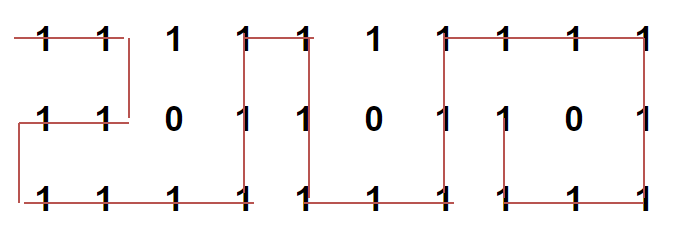Related Articles

# Longest Possible Route in a Matrix with Hurdles

• Difficulty Level : Medium
• Last Updated : 01 Jun, 2021

Given an M x N matrix, with a few hurdles arbitrarily placed, calculate the length of longest possible route possible from source to destination within the matrix. We are allowed to move to only adjacent cells which are not hurdles. The route cannot contains any diagonal moves and a location once visited in a particular path cannot be visited again.
For example, longest path with no hurdles from source to destination is highlighted for below matrix. The length of the path is 24.The idea is to use Backtracking. We start from the source cell of the matrix, move forward in all four allowed directions and recursively checks if they leads to the solution or not. If destination is found, we update the value of longest path else if none of the above solutions work we return false from our function.
Below is C++ implementation of above idea –

## CPP

 `// C++ program to find Longest Possible Route in a``// matrix with hurdles``#include ``using` `namespace` `std;``#define R 3``#define C 10` `// A Pair to store status of a cell. found is set to``// true of destination is reachable and value stores``// distance of longest path``struct` `Pair``{``    ``// true if destination is found``    ``bool` `found;` `    ``// stores cost of longest path from current cell to``    ``// destination cell``    ``int` `value;``};` `// Function to find Longest Possible Route in the``// matrix with hurdles. If the destination is not reachable``// the function returns false with cost INT_MAX.``// (i, j) is source cell and (x, y) is destination cell.``Pair findLongestPathUtil(``int` `mat[R][C], ``int` `i, ``int` `j,``    ``int` `x, ``int` `y, ``bool` `visited[R][C])``{` `    ``// if (i, j) itself is destination, return true``    ``if` `(i == x && j == y)``    ``{``        ``Pair p = { ``true``, 0 };``        ``return` `p;``    ``}` `    ``// if not a valid cell, return false``    ``if` `(i < 0 || i >= R || j < 0 || j >= C ||``            ``mat[i][j] == 0 || visited[i][j])``    ``{``        ``Pair p = { ``false``, INT_MAX };``        ``return` `p;``    ``}` `    ``// include (i, j) in current path i.e.``    ``// set visited(i, j) to true``    ``visited[i][j] = ``true``;` `    ``// res stores longest path from current cell (i, j) to``    ``// destination cell (x, y)``    ``int` `res = INT_MIN;` `    ``// go left from current cell``    ``Pair sol = findLongestPathUtil(mat, i, j - 1, x, y, visited);` `    ``// if destination can be reached on going left from current``    ``// cell, update res``    ``if` `(sol.found)``        ``res = max(res, sol.value);` `    ``// go right from current cell``    ``sol = findLongestPathUtil(mat, i, j + 1, x, y, visited);` `    ``// if destination can be reached on going right from current``    ``// cell, update res``    ``if` `(sol.found)``        ``res = max(res, sol.value);` `    ``// go up from current cell``    ``sol = findLongestPathUtil(mat, i - 1, j, x, y, visited);` `    ``// if destination can be reached on going up from current``    ``// cell, update res``    ``if` `(sol.found)``        ``res = max(res, sol.value);` `    ``// go down from current cell``    ``sol = findLongestPathUtil(mat, i + 1, j, x, y, visited);` `    ``// if destination can be reached on going down from current``    ``// cell, update res``    ``if` `(sol.found)``        ``res = max(res, sol.value);` `    ``// Backtrack``    ``visited[i][j] = ``false``;` `    ``// if destination can be reached from current cell,``    ``// return true``    ``if` `(res != INT_MIN)``    ``{``        ``Pair p = { ``true``, 1 + res };``        ``return` `p;``    ``}` `    ``// if destination can't be reached from current cell,``    ``// return false``    ``else``    ``{``        ``Pair p = { ``false``, INT_MAX };``        ``return` `p;``    ``}``}` `// A wrapper function over findLongestPathUtil()``void` `findLongestPath(``int` `mat[R][C], ``int` `i, ``int` `j, ``int` `x,``                                                  ``int` `y)``{``    ``// create a boolean matrix to store info about``    ``// cells already visited in current route``    ``bool` `visited[R][C];` `    ``// initialize visited to false``    ``memset``(visited, ``false``, ``sizeof` `visited);` `    ``// find longest route from (i, j) to (x, y) and``    ``// print its maximum cost``    ``Pair p = findLongestPathUtil(mat, i, j, x, y, visited);``    ``if` `(p.found)``        ``cout << ``"Length of longest possible route is "``             ``<< p.value;` `    ``// If the destination is not reachable``    ``else``        ``cout << ``"Destination not reachable from given source"``;``}` `// Driver code``int` `main()``{``    ``// input matrix with hurdles shown with number 0``    ``int` `mat[R][C] =``    ``{``        ``{ 1, 1, 1, 1, 1, 1, 1, 1, 1, 1 },``        ``{ 1, 1, 0, 1, 1, 0, 1, 1, 0, 1 },``        ``{ 1, 1, 1, 1, 1, 1, 1, 1, 1, 1 }``    ``};` `    ``// find longest path with source (0, 0) and``    ``// destination (1, 7)``    ``findLongestPath(mat, 0, 0, 1, 7);` `    ``return` `0;``}`

Output:

`Length of longest possible route is 24`